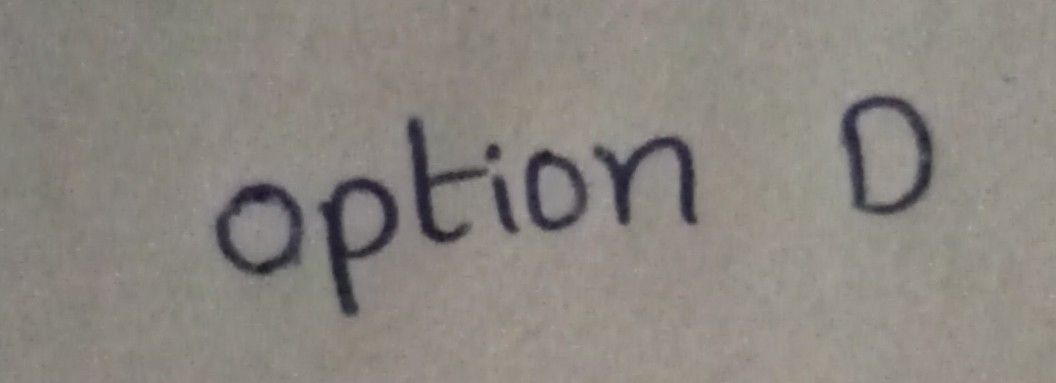Symbol
Problem2.The algebraic expression $3x+2y+6$ is $a$ A.Monomial B.Binomial C.Trinomial D.None of the above
7th-9th grade
Geometry
Search count: 105
SolutionQanda teacher - vinod